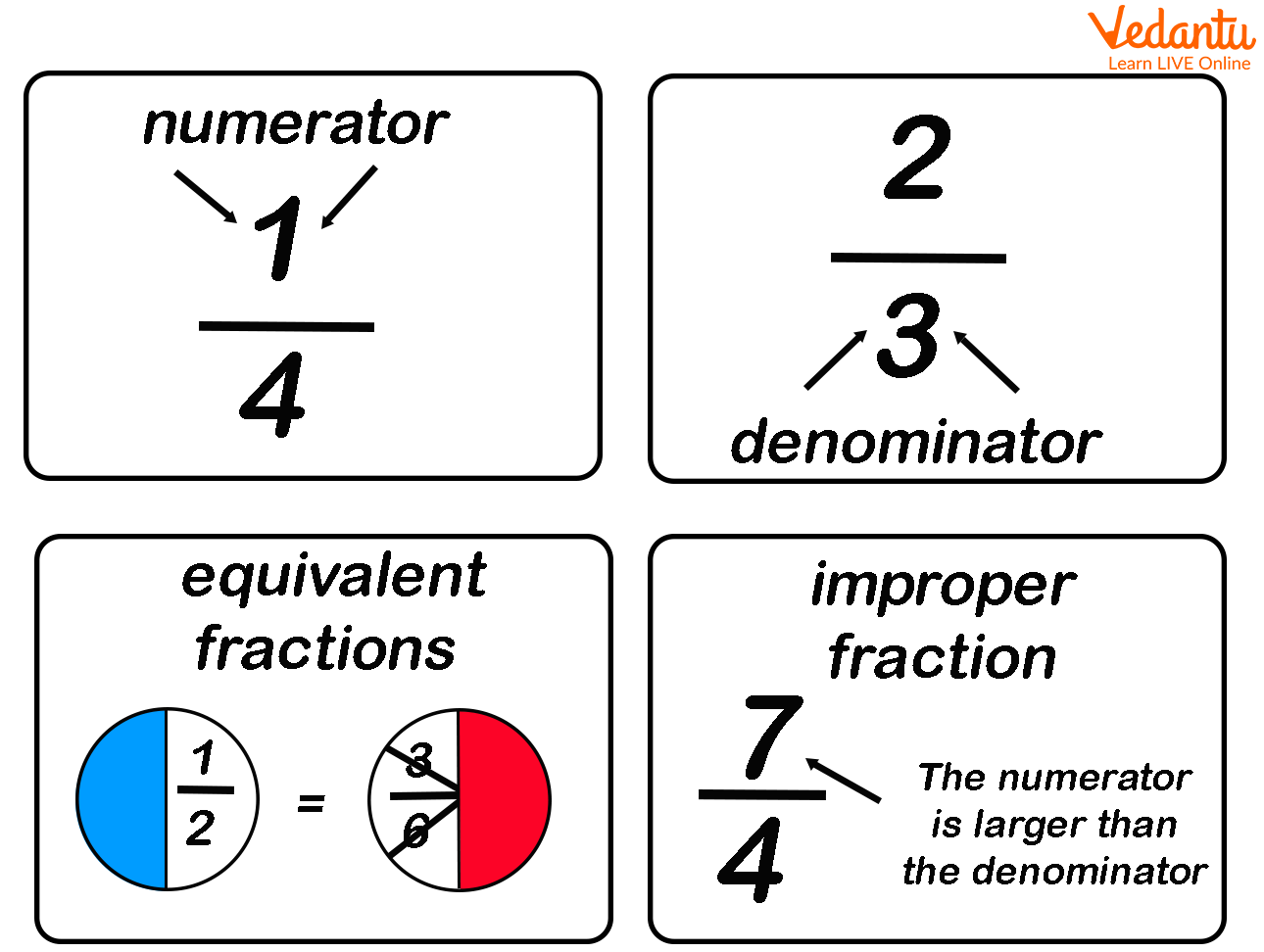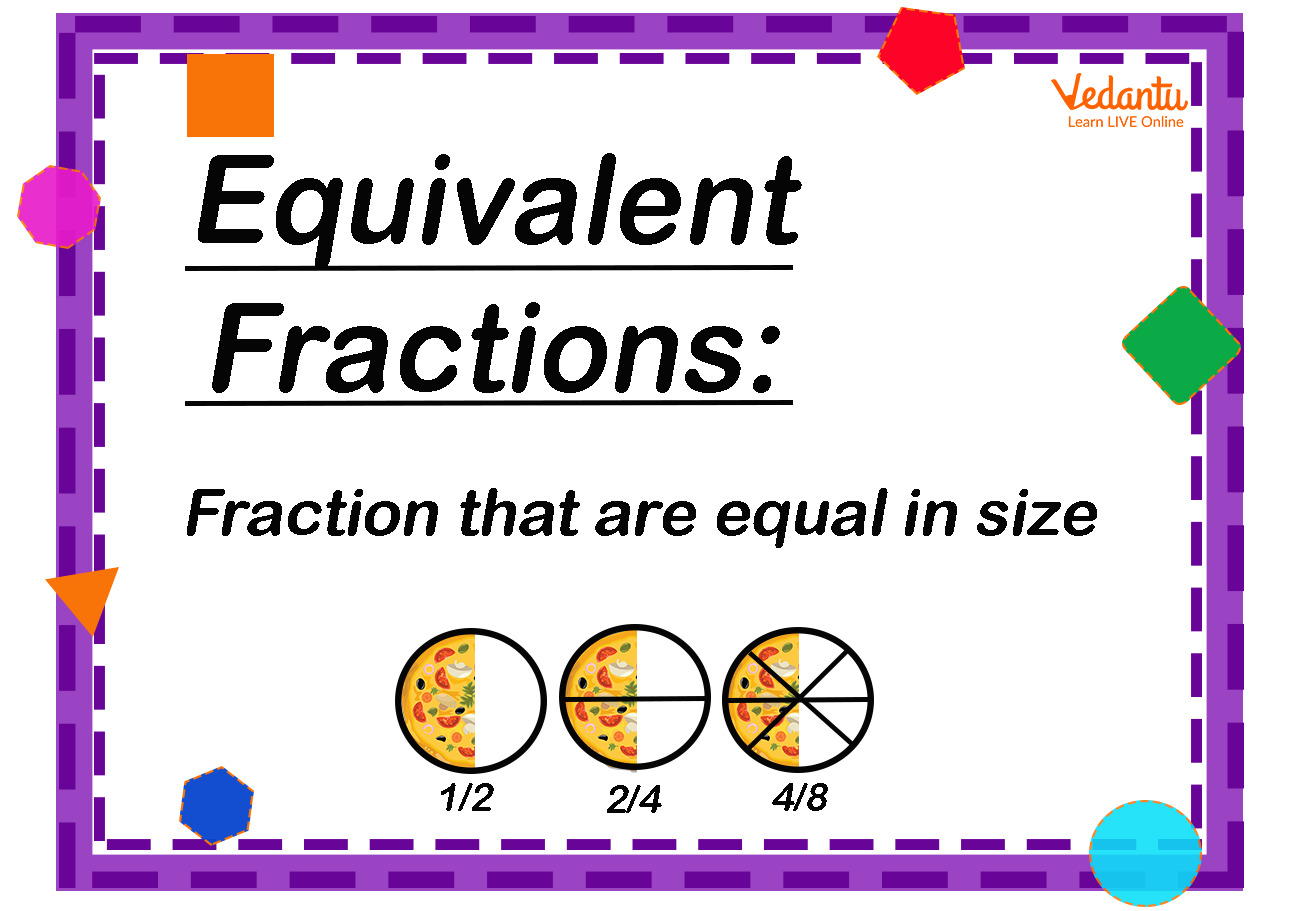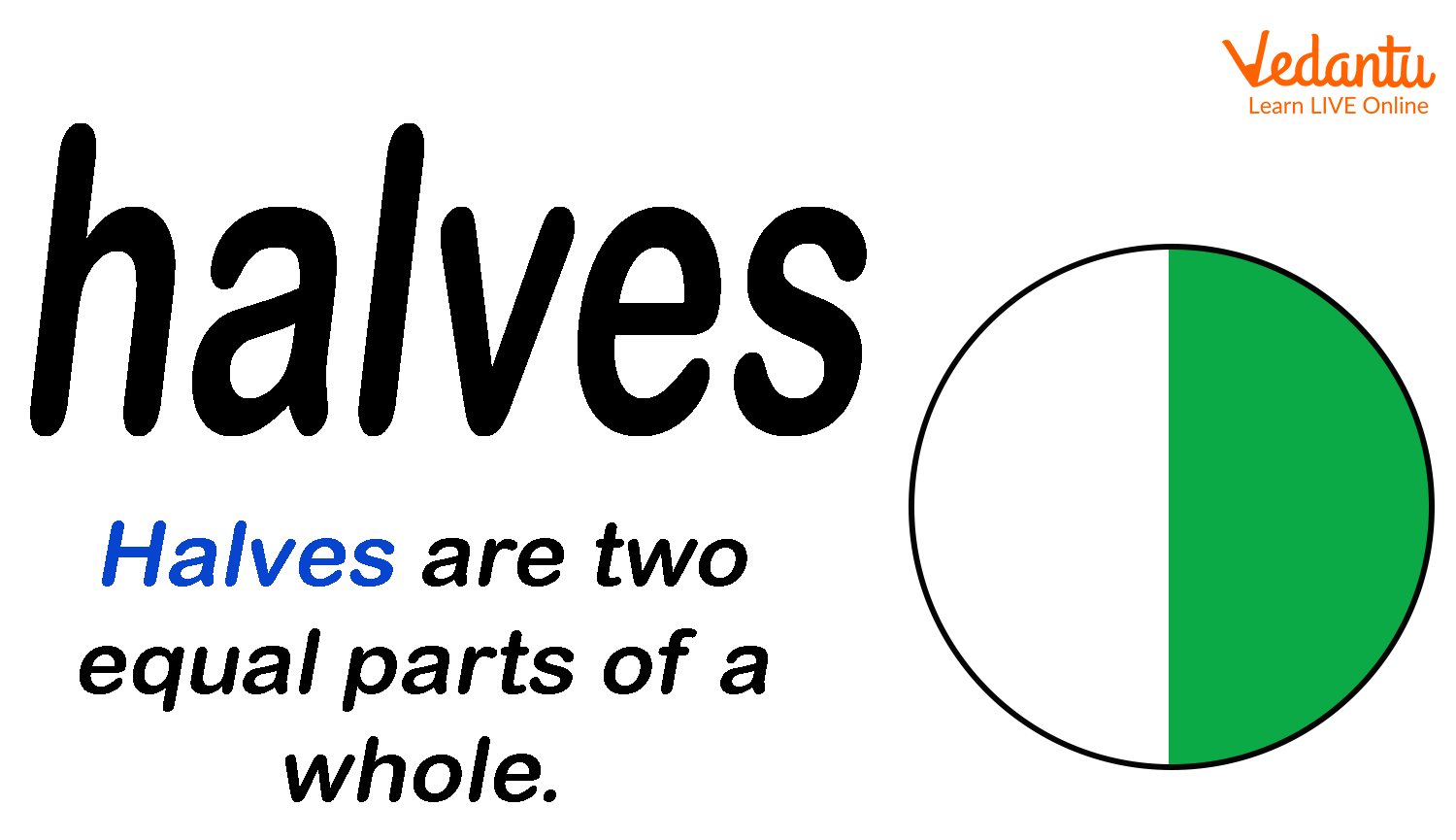Courses
Courses for Kids
Free study material
Free LIVE classes
More

# Fraction Vocabulary with List of Words## An Introduction to the Fraction Vocabulary

We know that fraction is part of something which is whole. This whole can be any object or number. Hence in general we can say that fraction is a small portion or part of any item. In the same way in terms of numbers, it is part of a whole number. There are a lot of terminologies are related to fractions which are often used in mathematics. These words come under fraction vocabulary. Some of the important words of fraction vocabulary are discussed below with their meanings.

### List of Words

A list of words of fraction vocabulary with their meaning are given below. You can use these words while writing or talking about fractions.

 S.No. Words 1 Fraction 2 Numerator 3 Denominator 4 Proper Fraction 5 Improper Fraction 6 Equivalent Fraction 7 Simplest Terms 8 Mixed Numbers 9 Half of 10 Quarter of

Let’s understand the meaning of these terms in detail.

1. Fraction – A fraction is a part of a collection, set or a whole.

2. Numerator – Numerator is the number or digits placed above the bar in a fraction, it represents how many equal parts of the whole are indicated.

3. Denominator – Denominator is the number or digits placed below the bar in a fraction. It represents how many equal parts are present in the whole.Fraction Vocabulary

1. Proper fraction – A proper fraction is a type of fraction where the numerator is less than the denominator, less than a whole

2. Improper fraction – An improper fraction is a type fraction where the numerator is greater than the denominator, greater than a whole

3. Equivalent fraction – Equivalent fraction is fractions that give equal fraction values. Hence equivalent fractions that name the same amount.Equivalent Fractions

1. Simplest terms – The simplest term is a fraction where the numerator number and the denominator have no common factors except 1. Hence the number 1 is the only common factor in such type of traction. For example, $\frac{2}{3}$ is the simplest term because there is no common factor except 1.

2. Mixed Number – A mixed number is consist of two parts. These are; a whole number and a fraction. For example 5 $\frac{1}{4}$ . Here 5 is the whole number while $\frac{1}{4}$ is a fractions. So this is a mixed number.

3. Half of- Half of is equal to$\frac{1}{2}$ .Half of

1. A quarter of- Quarter of is equal to $\frac{1}{4}$ .

### Conclusion

The above article explains some words of fraction vocabulary. These words are important for us to understand the concept of fractions. When we will go to the higher class it is important to clear the concept and terminologies of the previous class and this article will help students in doing so.

Last updated date: 20th Sep 2023
Total views: 136.5k
Views today: 2.36k

## FAQs on Fraction Vocabulary with List of Words

1. What are parts of fractions?

A fraction is consist of two parts. These are the numerator and the denominator. The number of digits placed on the top of the line is called the numerator. It indicates the number of parts of the whole or collection. The number or digits that are placed below the line is known as the denominator. For example, $\frac{3}{4}$ is a fraction where 3 is the numerator and 4 is the denominator.

2. Where do we see fractions in real life?

Fractions are an important part of our daily life because we are able to know what portion of a whole is required, have, or want. The real-life example of uses of fractions is used in baking, cooking, shopping etc. It is used in order to tell time as each minute is a fraction of the hour. When we go to buy household stuff or cooking materials things are often sold in fractions.